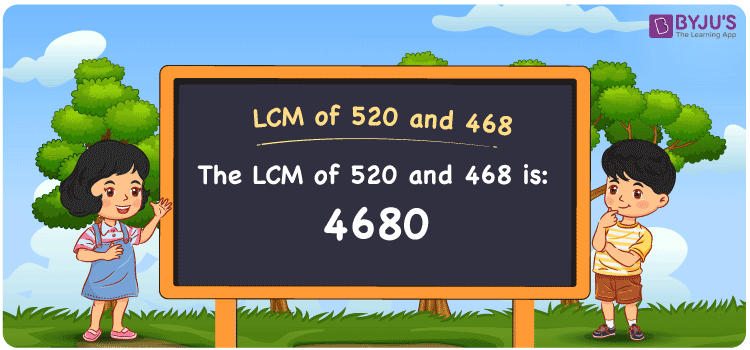# LCM of 520 and 468

LCM of 520 and 468 is 4680. The least common multiple is defined as the smallest common multiple of two or more numbers. The article Least Common Multiple (LCM) is designed by experts with the aim to provide the best study resource so that students grasp the LCM concept thoroughly. The detailed explanation of the LCM concept in simple language helps students to calculate the least common multiple of given numbers with ease. Let us learn in detail how to find the least common multiple of 520 and 468 in this article.

## What is LCM of 520 and 468?

The Least Common Multiple of 520 and 468 is 4680.## How to Find LCM of 520 and 468?

LCM of 520 and 468 can be determined using three methods

• Prime Factorisation
• Division method
• Listing the multiples

### LCM of 520 and 468 Using Prime Factorisation Method

In this method, the numbers can be expressed as the product of prime numbers. Hence, the numbers 520 and 468 can be expressed as shown below;

520 = 2 × 2 × 2 × 5 × 13

468 = 2 × 2 × 3 × 3 × 13

LCM (520, 468) = 2 × 2 × 2 × 3 × 3 × 5 × 13 = 4680

### LCM of 520 and 468 Using Division Method

In the division method, we divide the numbers 520 and 468 by their prime factors until we get the result as one in the complete row to find their LCM. The product of these divisors represents the least common multiple of 520 and 468.

 2 520 468 2 260 234 2 130 117 3 65 117 3 65 39 5 65 13 13 13 13 x 1 1

No more further division can be done.

Hence, LCM (520, 468) = 2 × 2 × 2 × 3 × 3 × 5 × 13 = 4680

### LCM of 520 and 468 Using Listing the Multiples

In this method, we list out the multiples of 520 and 468 to calculate their LCM. The below table shows the multiples of 520 and 468.

 Multiples of 520 Multiples of 468 520 468 1040 936 1560 1404 2080 1872 2600 2340 3120 2808 3640 3276 4160 3744 4680 4212 5200 4680

LCM (520, 468) = 4680

## Related Articles

LCM of Two Numbers

LCM Calculator

Prime Factorization and Division Method for LCM and HCF

LCM Formula

LCM with Examples

HCF and LCM

## Video Lesson on Applications of LCM## Solved Examples

Q. 1: What is the smallest number that is divisible by both 520 and 468?

Solution: 4680 is the smallest number that is divisible by both 520 and 468.

Q. 2: The GCD and LCM of the two numbers are 52 and 4680. If one number is 468, calculate the other number.

Solution: Let the other number be q

We know that

(GCD × LCM) = 468 × q

q = (GCD × LCM) / 468

q = (52 × 4680) / 468

q = 520

Hence the other number is 520.

## Frequently Asked Questions on LCM of 520 and 468

### What is the LCM of 520 and 468?

The LCM of 520 and 468 is 4680.

### Is the LCM of 520 and 468 the same as the HCF of 520 and 468?

No. The LCM of 520 and 468 is 4680 and the HCF of 520 and 468 is 52.

### Is 4680 the LCM of 520 and 468?

Yes. The LCM of 520 and 468 is 4680.

### How to calculate the LCM of 520 and 468 using Prime Factorisation?

520 = 2 × 2 × 2 × 5 × 13

468 = 2 × 2 × 3 × 3 × 13

LCM (520, 468) = 2 × 2 × 2 × 3 × 3 × 5 × 13 = 4680

Hence the LCM of 520 and 468 is 4680

### Which of the following is the LCM of 520 and 468? 3000, 2900, 4680, 1000

The LCM of 520 and 468 is 4680.